### Keyword NMR

This keyword activates the calculation of closed-shell NMR shielding tensors.
Options:
 NICS Calculation of nuclear-independent chemical shifts. READ Specifies that a list of nuclei for shielding tensor calculations is read from the keyword body of NMR. SPINROT Calculation of the Nuclear Spin-Rotation Constants (NSRC).
Description:
The magnetic shielding tensors can be calculated either within the AUXIS or BASIS GIAO formalism . One or the other formalism is activated by the AUXIS or BASIS options of the VXCTYPE keyword. The GIAO scheme implemented thereby can be combined with ECPs and MCPs on neighboring centers in order to include scalar relativistic effects in the magnetic shielding tensor calculation. The default output only shows the calculated isotropic magnetic shielding [ppm] of each nucleus in the system: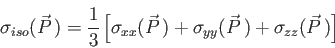(28)

To print the full shielding tensor, PRINT NMR must be used (see 4.12.2). The user can select individual atoms by the atomic symbol (e.g. H4) or atom groups by the element symbols (e.g. H) for which magnetic shielding tensors will be calculated with the READ option. In the following example, only the shielding tensors of the hydrogen atoms in NH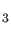are calculated.

 NMR READ
H
SYMMETRY ON
BASIS (IGLO-III)
VXCTYPE BASIS PBE
#
GEOMETRY Z-MATRIX ANGSTROM
N
H1      1  rNH
H2      1  rNH        2  aHNH
H3      1  rNH        3  aHNH       2  wHHNH
#
VARIABLES
rNH     1.01700
aHNH  107.80000
wHHNH 120.00000


The NICS option of the NMR keyword  activates shielding tensor calculations at the position of dummy atoms as specified in the keyword body of the GEOMETRY keyword. The following input example enables NICS calculations at two different positions, specified by X1 and X2, in an Al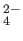cluster.

 NMR NICS READ
X1 X2
CHARGE -2
PRINT NMR
BASIS (DZVP)
VXCTYPE BLYP
AUXIS (GEN-A2)
SCFTYP TOL=1.E-8 CDF=1.E-5
#
GEOMETRY Z-MATRIX ANGSTROM
X1
Al       1  AlX2
Al       1  AlX3        2  AlXAl3
Al       1  AlX4        2  AlXAl4       3  DIH4
Al       1  AlX5        3  AlXAl5       2  DIH5
X2       1  1.25        2  90.0         3  90.0
#
VARIABLES
AlX2        1.831000
AlX3        1.831000
AlX4        1.831000
AlX5        1.831000
AlXAl3     90.000000
AlXAl4     90.000000
AlXAl5     90.000000
DIH4      180.000000
DIH5      180.000000


The simulation of the magnetic shieldings along a BOMD trajectory is calculated in two steps as discussed in Section 4.7.3. A BOMD magnetic shielding calculation is activated by the option NMR=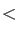Integerof the simulation keyword (see 4.7.3). HereInteger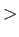specifies the number of the atom for which the trajectory data are printed. The user can select the nuclei by activating the option READ of the NMR keyword (see 4.8.8), otherwise the magnetic shielding of all nuclei will be calculated along the BOMD trajectory. In the following example, the magnetic shielding of the carbon nucleus of methanol along a BOMD trajectory is calculated as:

 TITLE METHANOL MD SIMULATION
#
C
BASIS (AUG-CC-PVDZ)
VXCTYPE AUXIS PW91
TRAJECTORY RESTART PART=10000-110000
SIMULATE CALCULATE NMR=1 INT=25
#
GEOMETRY Z-MATRIX
C
H   1 HC2
H   1 HC3      2 HCH3
H   1 HC4      3 HCH4     2 DIH4
O   1 OC5      3 OCH5     2 DIH5
H   5 HO6      1 HOC6     3 DIH6
VARIABLES
HC2       1.098100
HC3       1.098100
HCH3      109.120
HC4       1.098100
HCH4      109.120
DIH4      120.000
OC5       1.429200
OCH5      109.471
DIH5      240.000
HO6       0.975100
HOC6      107.690
DIH6      180.000
END


At the end of the corresponding output, the average and the standard deviation of the isotropic magnetic shieldings of all selected nuclei are printed.

The SPINROT option activates the computation of the NSRC for all magnetically active nuclei in the molecule . For the NSRC, it is necessary to indicate the isotopic composition of the molecule which can be specified by the nuclear masses in the GEOMETRY definition. The NSR tensor is evaluated in the principal axes of the tensor of inertia. The output gives the values of the diagonal elements of the NSR tensor,,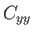and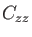which are associated with the moments of inertia arranged in the order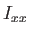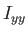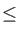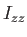. The components are labeled A, B and C. Also the isotropic and anisotropic NSRC are given in the output. The isotropic NSRC is calculated as: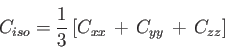(29)

The corresponding anisotropic NSRC is calculated from the eigenvalues of the traceless NSR tensor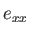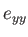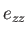as: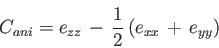(30)

The NSRC can also be calculated along a BOMD trajectory. The example given below shows the input for such a calculation of the acetylene-HF complex, the H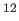C-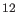C-DF isotopologue. Note that the SIMULATION keyword options for the NSRC calculation are the same as for the magnetic shielding calculation. The NSRC calculation is triggered by the NMR SPINROT keyword line.

 DISPERSION
BASIS (AUG-CC-PVDZ)
AUXIS (GEN-A2)
SCFTYPE MAX=1000 TOL=0.100E-04
VXCTYPE OPTX-PBE AUXIS
TRAJECTORY RESTART PART=10000-12000 INT=20
NMR SPINROT
SIMULATION CALCULATE NMR=1 INT=20
#
# Cartesian coordinates of MD step 410000
#
GEOMETRY CARTESIAN ANGSTROM
F   -66.916797   19.630729  -13.088069      18.998
H   -67.101518   18.720636  -12.997091       2.014
C    54.166066  -15.936748    9.958016      12.000
C    53.957601  -15.619981   11.173715      12.000
H    54.766661  -16.089846    9.024499       1.008
H    53.312450  -15.623649   12.049412       1.008


In the output,

                    NSR CONSTANTS [kHz] FOR ATOM 1

TIME [FS]       XX       YY       ZZ      C_K      <C_K>

5000.0       1.82     2.33     2.06     2.07     2.07
5010.0       2.76     1.91     1.87     2.18     2.12
5020.0       2.42     1.60     1.71     1.91     2.05
5030.0       1.63     1.77     1.90     1.77     1.98
5040.0       0.61     2.30     2.33     1.75     1.93
5050.0       0.04     2.87     2.83     1.91     1.93
5060.0       1.23     3.17     3.30     2.57     2.02
5070.0       2.32     2.91     3.31     2.85     2.12
5080.0       1.71     2.69     3.23     2.54     2.17
:            :        :        :        :        :

*** NMR SHIELDINGS STATISTIC ***

CHEMICAL SHIELDING FOR ATOM 1 ( F )
AVERAGE SHIELDING [ppm] =   380.82
STD DEV SHIELDING [ppm] =    20.27

CHEMICAL SHIELDING FOR ATOM 2 ( H )
AVERAGE SHIELDING [ppm] =    28.38
STD DEV SHIELDING [ppm] =     1.53

:   :      :           :       :

*** NSR CONSTANTS STATISTIC ***

NSR CONSTANT FOR ATOM 1 ( F )

AVERAGE NSR XX-CONSTANT [kHz] =     3.80
STD DEV NSR XX-CONSTANT [kHz] =     3.63
AVERAGE NSR YY-CONSTANT [kHz] =     2.91
STD DEV NSR YY-CONSTANT [kHz] =     0.99
AVERAGE NSR ZZ-CONSTANT [kHz] =     3.15
STD DEV NSR ZZ-CONSTANT [kHz] =     1.34

NSR CONSTANT FOR ATOM 2 ( H )

AVERAGE NSR XX-CONSTANT [kHz] =    -0.12
STD DEV NSR XX-CONSTANT [kHz] =     0.12
AVERAGE NSR YY-CONSTANT [kHz] =    -0.06
STD DEV NSR YY-CONSTANT [kHz] =     0.01
AVERAGE NSR ZZ-CONSTANT [kHz] =    -0.06
STD DEV NSR ZZ-CONSTANT [kHz] =     0.01

:   :   :        :           :       :


the instantaneous NSR tensor diagonal elements and isotropic value along with its average are listed for the selected NMR atom in the SIMULATION keyword line. The NSR constant statistics at the end of the output are given for all atoms. Note also the NMR shielding statistics. Thus, NSR constants and NMR shieldings are always calculated together.